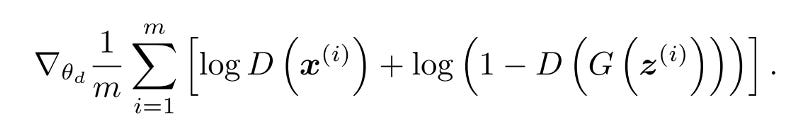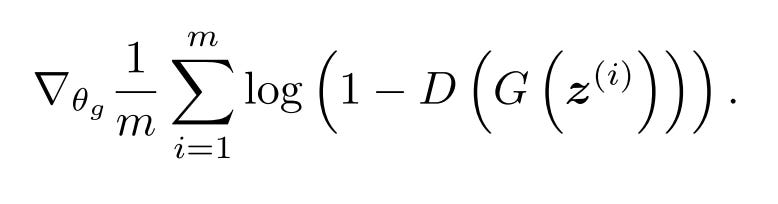# Introduction to CycleGANs

In this blog post, we will explore a cutting edge Deep Learning Algorithm Cycle Generative Adversarial Networks (CycleGAN). But before heading on, let’s first look at the results it has been able to achieve.

If that’s not enough to blow away your mind, I don’t know what is. Now that you have developed interest in the topic, let’s start with the same.

### Brief Introduction to GAN

“The coolest idea in deep learning in the last 20 years.” — Yann LeCun on GANs.

GANs belong to the set of algorithms named generative models. These algorithms belong to the field of unsupervised learning, a sub-set of ML which aims to study algorithms that learn the underlying structure of the given data, without specifying a target value.

Generative Adversarial Networks are composed of two models:

• The first model is called a Generator and it aims to generate new data similar to the expected one. The Generator could be asimilated to a human art forger, which creates fake works of art.
• The second model is named the Discriminator. This model’s goal is to recognize if an input data is ‘real’ — belongs to the original dataset — or if it is ‘fake’ — generated by a forger. In this scenario, a Discriminator is analogous to the police (or an art expert), which tries to detect artworks as truthful or fraud.

The Loss Equations for GANs are given as :The gradient ascent expression for the discriminator. The first term corresponds to optimizing the probability that the real data (x) is rated highly. The second term corresponds to optimizing the probability that the generated data G(z) is rated poorly. Notice we apply the gradient to the discriminator, not the generator.The gradient descent expression for the generator. The term corresponds to optimizing the probability that the generated data G(z) is rated highly. Notice we apply the gradient to the generator network, not the discriminator.

### CycleGAN

After seeing the horse2zebra gif above, most of you would be thinking of a following approach : Prepare a dataset of Horses and Zebras in the same environment, in exactly the same locations and then create some kind of a mapping between the two with the help of a Neural Network. But that’s not how it works because it would be close to impossible to get such a dataset. The beauty of the algorithm lies in achieving the same result in a smart and easy way with a dataset containing just the images of Horses and Zebras.

### Architecture

It consists of :

• Two mappings G : X -> Y and F : Y -> X
• Corresponding Adversarial discriminators Dx and Dy

Role of G: G is trying to translate X into outputs, which are fed through Dy to check whether they are real or fake according to Domain Y

Role of F : F is trying to translate Y into outputs, which are fed through Dx to check if they are indistinguishable from Domain X

### Loss Functions

The real power of CycleGANs lie in the loss functions used by it. In addition to the Generator and Discriminator loss ( as described above ) it involves one more type of loss given by :

### Cyclic-Consistency Loss

This kind of loss uses the intuition that if we translate a sample from Domain X to Y using mapping function G and then map it back to X using function F, how close are we from arriving at the original sample. Similarly, it calculates the loss incurred by translating a sample from Y to X and then back again to Y. This cyclic loss should be minimised.

`g_loss_G_cycle = tf.reduce_mean(tf.abs(real_X — genF_back)) + tf.reduce_mean(tf.abs(real_Y — genG_back))`
`g_loss_F_cycle = tf.reduce_mean(tf.abs(real_X — genF_back)) + tf.reduce_mean(tf.abs(real_Y — genG_back))`

### Total Loss

Total generator loss is given as :

g_loss_G = g_loss_G_disc + lambda * g_loss_G_cycle

g_loss_F = g_loss_F_disc + lambda * g_loss_F_cycle

Here, g_loss_G_disc & g_loss_F_disc are Generator Losses, so that the generator is able to generate fake images, which discriminator identifies as real ones.

Apparently, Cyclic Losses are so important that they are multiplied by a constant lambda (in the paper the value 10 was used)

The Total Discriminator loss is same as that of simple GANs :

`Gz = generator(z_in) #Generates images from random z vectors (noise) Dx = discriminator(real_in) #Produces probabilities for real imagesDg = discriminator(Gz) #Produces probabilities for generator images#These functions together define the optimization objective of the GAN.d_loss = -tf.reduce_mean(tf.log(Dx) + tf.log(1.-Dg)) #This optimizes the discriminator.`

Source: Deep Learning on Medium